Enhanced OWA

Some years ago Jim Breakall, WA3FET, devised the Optimized Wideband Antenna. It is a Yagi with close-spaced first director that provides low SWR over a wide bandwidth with direct 50Ω feed. Although the OWA has less forward gain than a conventional design with matching network, its wide SWR bandwidth and feed simplicity make it popular.

Bending the OWA driven element can improve forward gain while retaining wide SWR bandwidth and direct feed.

40 Meters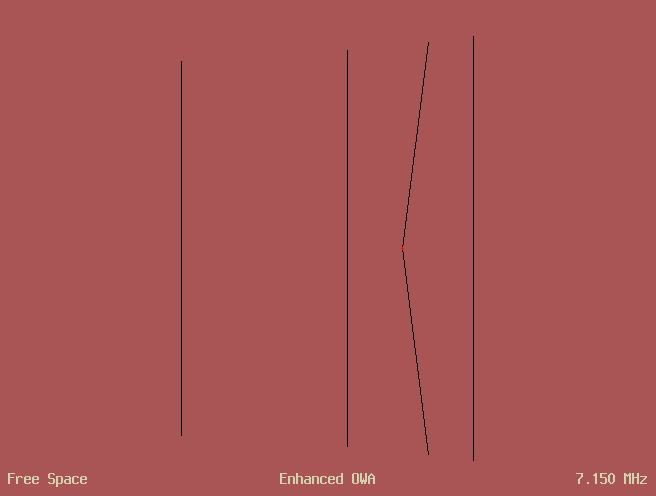I used AO 9.66 to maximize forward gain for a 4-element OWA on a 570″ boom with F/R no less than 22 dB and SWR no greater than 1.4 over 7.0 to 7.3 MHz. The blue curves show the response. Then I let the driven element bend in the center and reoptimized with the same constraints. The red curves are for a 7.2° bend. Bending additional elements can provide up to 0.3 dB more gain.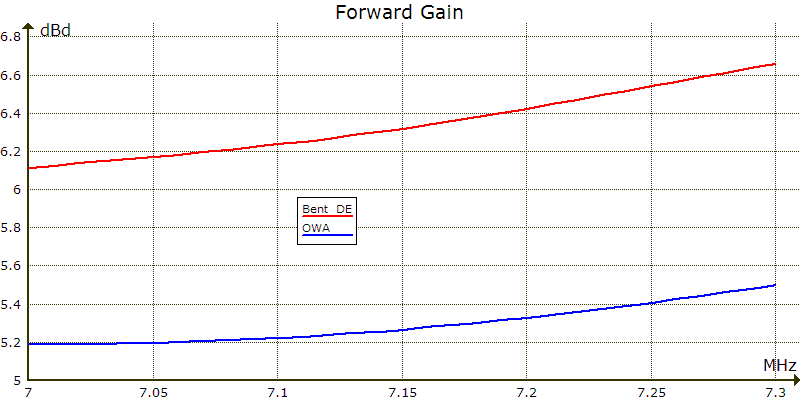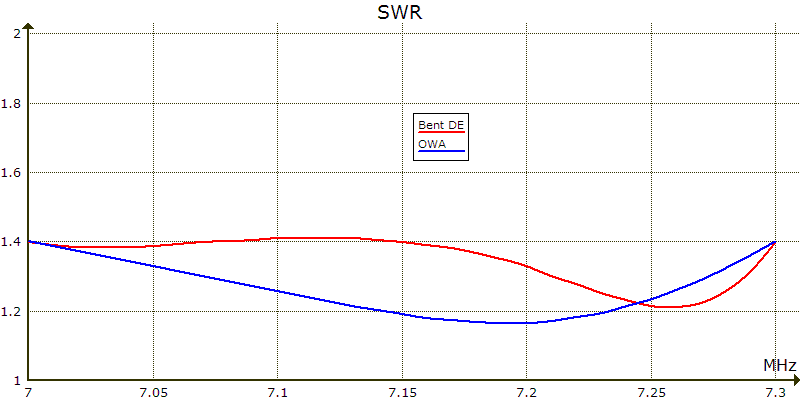To explore the gain/pattern trade-off, I reoptimized with maximum SWR of 1.4 and varied minimum F/R.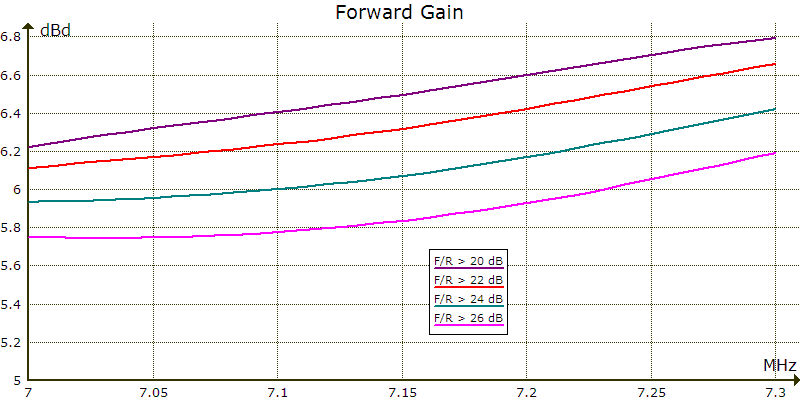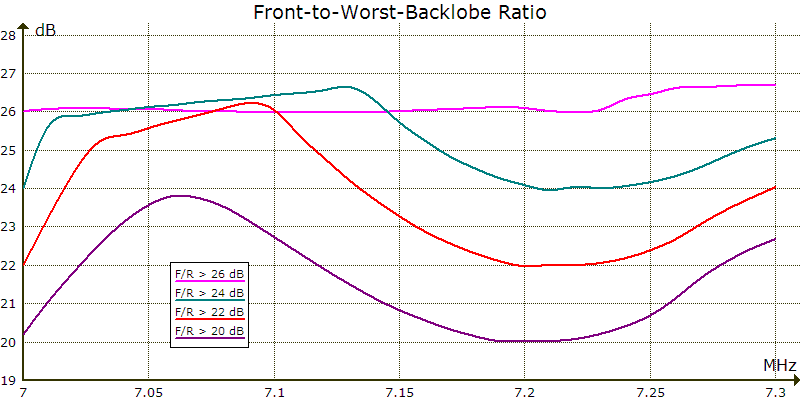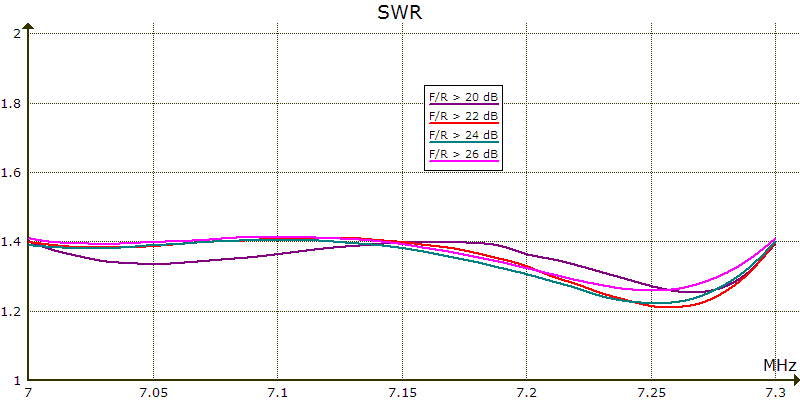To explore the gain/SWR trade-off, I reoptimized with minimum F/R of 22 dB and varied maximum SWR.Narrowband Pattern

Some OWAs are designed for a good pattern at the low end of the band but allow it to degrade higher up where it may not be needed. Relaxing F/R bandwidth yields much higher forward gain. A bent driven element offers less advantage for these designs. The dashed curves below are for OWAs with narrowband F/R. The solid curves, from above, are for wideband F/R.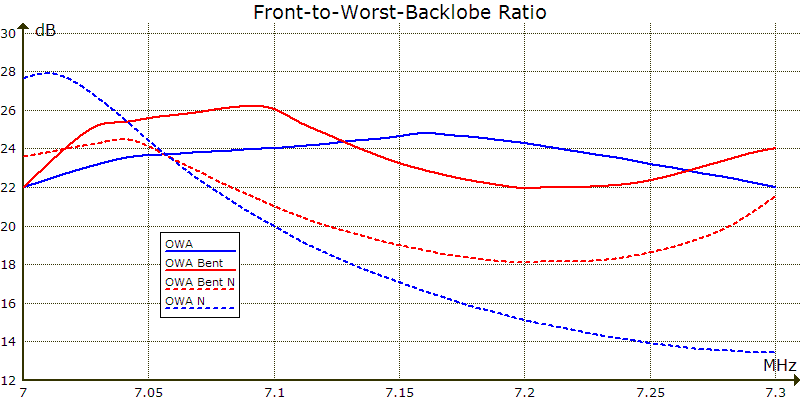Antenna Files

Enhanced OWA
Free Space Symmetric
7 7.075 7.15 7.225 7.3 MHz
4 6061-T6 wires, inches
ang = -7.20994
x1 = 0
x2 = 138.63
x3 = 245.5951
x4 = 570
y1 = 413.1038
y2 = 404.9571
y3 = 386.8065
y4 = 365.7719
1  x1 0 0  x1 y1 0  1.4
rotate end1 z ang
1  x2 0 0  x2 y2 0  1.4
rotate end
1  x3 0 0  x3 y3 0  1.4
1  x4 0 0  x4 y4 0  1.4
1 source
Wire 2, end1

Enhanced OWA - Narrowband F/R
Free Space Symmetric
7 7.1 7.3 MHz
4 6061-T6 wires, inches
ang = -8.210171
x1 = 0
x2 = 156.1316
x3 = 275.1442
x4 = 570
y1 = 412.7051
y2 = 403.4081
y3 = 386.9267
y4 = 371.5446
1  x1 0 0  x1 y1 0  1.4
rotate end1 z ang
1  x2 0 0  x2 y2 0  1.4
rotate end
1  x3 0 0  x3 y3 0  1.4
1  x4 0 0  x4 y4 0  1.4
1 source
Wire 2, end1

15 Meters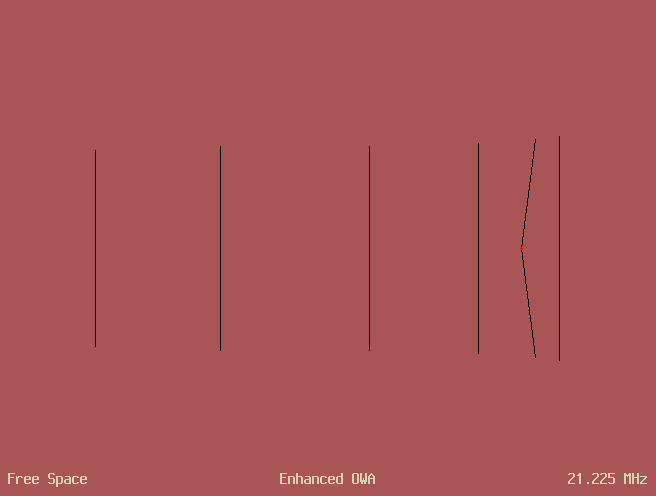I used AO 9.67 to maximize forward gain for a 6-element OWA on a 570″ boom with F/R no less than 22 dB and SWR no greater than 1.3 over 21.0 to 21.45 MHz. I repeated for 28 dB F/R. Then I let the driven element bend in the center and reoptimized with the same constraints.

The bent driven element improved forward gain just 0.2 dB at 22 dB F/R but 0.55–0.85 dB at 28 dB F/R. The enhanced OWA with 28 dB F/R has slightly more gain than the conventional OWA with 22 dB F/R.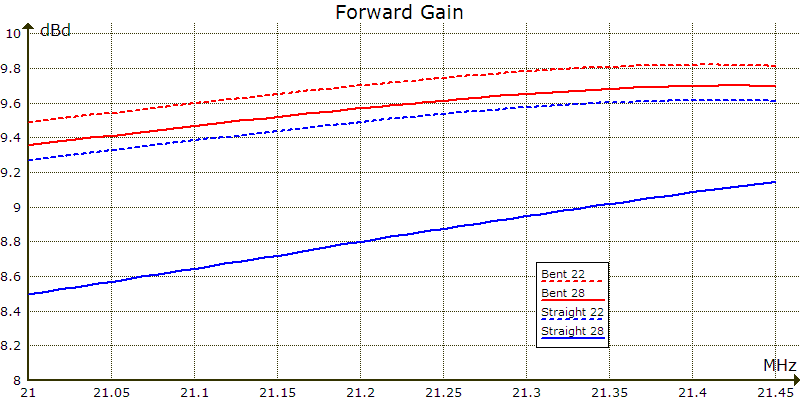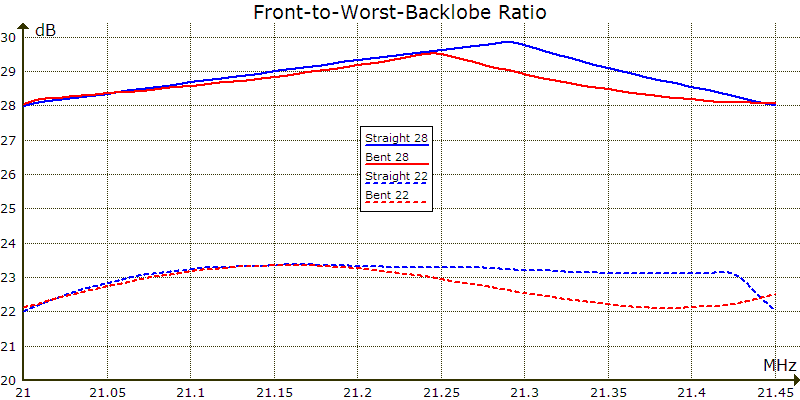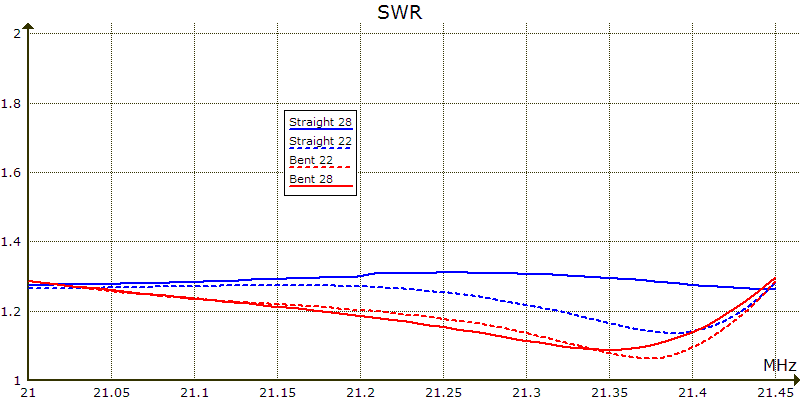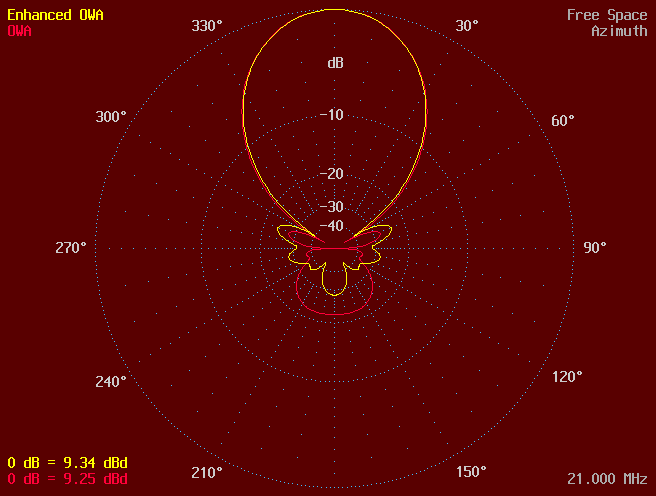Antenna Files

Enhanced OWA - 22 dB F/R minimum
Free Space Symmetric
21 21.2 21.37 21.45 MHz
6 6061-T6 wires, inches
ang = -6.403389
x1 = 0
x2 = 41.09755
x3 = 94.9448
x4 = 228.5655
x5 = 418.725
x6 = 570
y1 = 138.3705
y2 = 134.5689
y3 = 129.3241
y4 = 126.3879
y5 = 124.7475
y6 = 123.3673
1   x1  -y1   0   x1   y1   0   .84
rotate end1 z ang
1   x2    0   0   x2   y2   0   .84
rotate end
1   x3  -y3   0   x3   y3   0   .84
1   x4  -y4   0   x4   y4   0   .84
1   x5  -y5   0   x5   y5   0   .84
1   x6  -y6   0   x6   y6   0   .84
1 source
Wire 2, end1

Enhanced OWA - 28 dB F/R minimum
Free Space Symmetric
21 21.2 21.37 21.45 MHz
6 6061-T6 wires, inches
ang = -7.300423
x1 = 0
x2 = 45.73473
x3 = 98.67726
x4 = 232.771
x5 = 416.7147
x6 = 570
y1 = 138.2157
y2 = 134.8994
y3 = 129.3929
y4 = 125.8002
y5 = 125.1475
y6 = 120.4598
1   x1  -y1   0   x1   y1   0   .84
rotate end1 z ang
1   x2    0   0   x2   y2   0   .84
rotate end
1   x3  -y3   0   x3   y3   0   .84
1   x4  -y4   0   x4   y4   0   .84
1   x5  -y5   0   x5   y5   0   .84
1   x6  -y6   0   x6   y6   0   .84
1 source
Wire 2, end1

October 6, 2018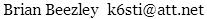88–108 MHz